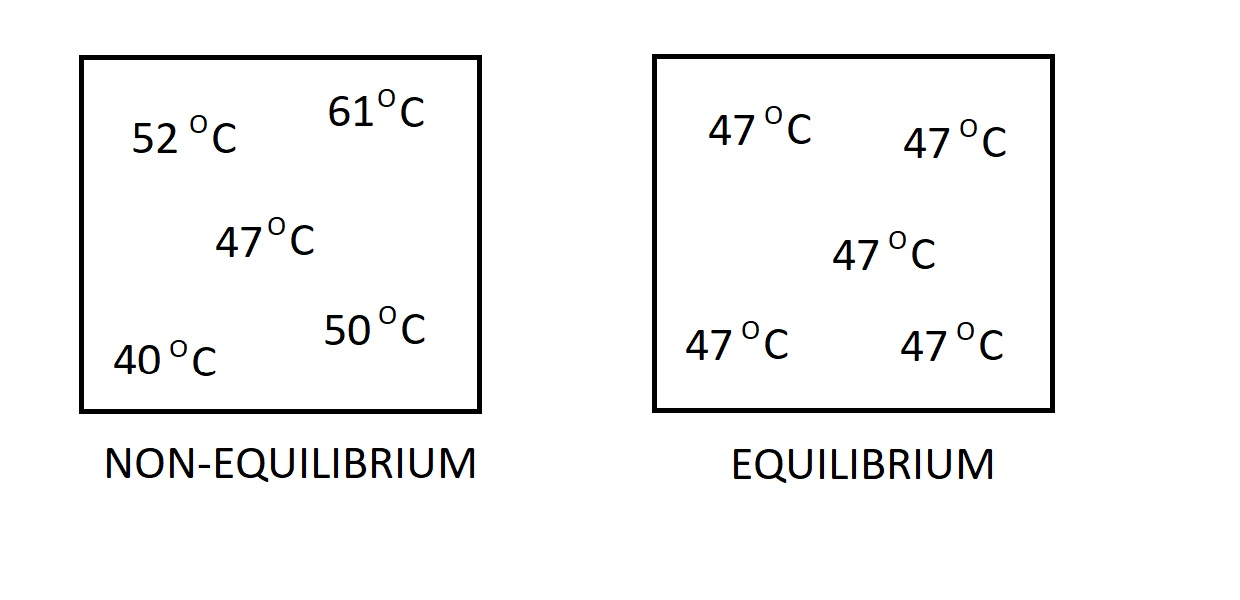# Equilibrium

When a system is in equilibrium it is in a state of balance.  This means that the system is not undergoing any changes and all points throughout the system should measure the same for all properties.  However, if the state of one property changes within the system, than the state of the whole system will change to reach a new equilibrium.

### Types of Equilibrium

When a system is in equilibrium there will be no unbalanced potentials within the system. In essence a system that is in equilibrium will experience no change when it is isolated from its surroundings.

There are many different types of equilibrium.  One type is thermal equilibrium.  When a system reaches this state the temperature through the system will be the same.  As a result, there will be no heat flow through the system.  This is because a temperature differential is the driving force for heat flow.Another type of equilibrium is mechanical.  In this case we are looking at the pressure within the system.  If there is no change in pressure in respect to time, than the system is in a state of mechanical equilibrium.  However, the pressure value inside the system are allowed to vary due to gravitational effects.

Another type is phase equilibrium.  This will occur when the mass of each phase reaches equilibrium and stays there.

Finally, another type of equilibrium is chemical.  This occurs when the chemical composition of the system doesn’t change with time.

### State Postulate

A system is described by a series of properties.  However,  to determine the state of the system we don’t need to know every single property.  Instead we only need to know the state of a select few properties.  From the state of these properties we can make an assumption for the remaining properties.  The number of properties required to make this assumption are called the state postulate.

At a minimum there must be two independent properties to specify a state postulate.   Hence, for two properties to be independent one property can be varied while the other remains fixed.  An example of two independent properties are temperature and specific volume.